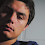## Wednesday, February 18, 2009

### Problem 12: Triangle, Cevian, Angles, Congruence

Proposed ProblemSee complete Problem 12 at:
http://gogeometry.com/problem/problem012.htm

Level: High School, SAT Prep, College geometry

1.Locate a point E on AC such that BC=CE. Join B to E. Now it's easy to see that AE=CD and angle AEB = 115 = angle BDC which makes BE=BD. Hence tringles ABE & CBD are SAS congruent; thus x=50.
Ajit: ajitathle@gmail.com

2.Denote angle ABD=α=115º-x. Suppose α>65º then AD>AB, and since AD=BC, BC>AB.Now in triangle ABC we get x>50º, that is 115º-α>50º, or α<65º in contradiction to our assumption α>65º. So α=65º and x=50º.

3.Solution by cristian to this problem:

http://youtu.be/mhh1YO0LLII

4.5.Since I am not able to see all links above, I post my solution, hoping it is not among the previous ones, although it is very simple: Take E - reflection of C in BD, DE=CD and easy angle chase gives <ADE=50, i.e. triangles ADE and BCD are congruent (s.a.s.), hence AE=BD and <DAE=15, therefore triangles ABE and BAD are congruent (s.s.s.) with <BAE=<ABD. From this we get x+15=115-x, or x=50.
Best regards,
Stan Fulger

6.7.This solution is clearer when illustrated, so grab a pen and a piece of paper.

Angle BDC = 180° - 50° - 15° = 115°
Angle ADB = 180° - 115° = 65°

Add point E to the figure and connect it to points A, B and E such that a duplicate of triangle BCD is formed with angle ADE = 15°. Now, we shall prove that point E sits on line AB.

Focus on triangle BDE. It is isosceles because line DE = line BD. Angle BDE = 65° - 15° = 50°. Angle BED = (180° - 50°)/2 = 65°. Angle AED = angle BDC = 115° because triangles ADE and BDC are identical.
Angle AED + angle BED = 180°, which is the angle measure of a straight line. Therefore, points A, E and B are collinear.

Since points A, E and B are collinear, angle BAC = angle EAC
So, angle BAC = angle BCD because triangles ADE and BCD are congruent.
Therefore, angle BAC = 50°

Angle x = 50°

8.Consider triangle ABC
sinx/BC=sin50/AB
BC=ABsinx/sin50

Consider triangle ABD

sinx/sin50=sin(115-x)/sin65
sin65/sin50/sin(115-x)/sinx
It is easy to see that x=50

1.A better solution after 1.5 years of thinking lol
<ABD=115-x
By considering triangle ABD with sine law
By considering triangle BCD with sine law
sin115/BC=sin50/BD
BC/BD=sin115/sin50---------(2)
Equating (1) & (2)
sin(115-x)/sinx=sin115/sin50
sin115sinx=sin(115-x)sin50
cos(115-x)-cos(115+x)=cos(65-x)-cos(165-x)
cos(115-x)+cos(65-x)=cos(65-x)-cos(165-x)
cos(115-x)=-cos(165-x)
cos(115-x)+cos(165-x)=0
2cos(140-x)cos50=0
cos(140-x)=0
140-x=90
x=50

9.E on AC such that BC=CE. So AE=CD and m(AEB)=m(CDB) and BE=CD => AEB is congruent to CDB => x=50 degrees.

Additionally E is the power of a point wrt circumcircle of BDC.